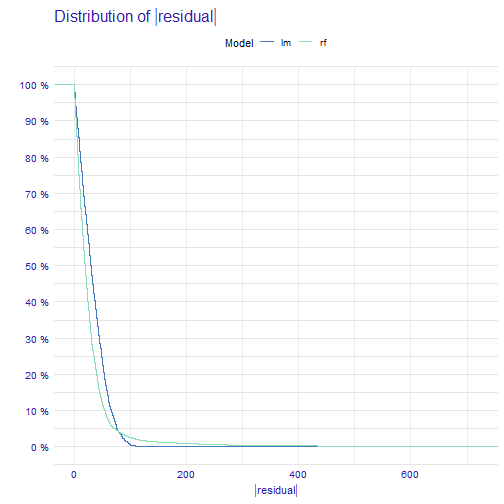## Data

To illustrate application of `auditor` we will use dataset “dragons” available in the `DALEX` package. The dataset contains characteristics of fictional creatures (dragons), like year of birth, height, weight, etc (see below). The goal is to predict the length of life of dragons (a regression problem).

``````library(DALEX)
data(dragons)
``````
``````##   year_of_birth   height   weight scars colour year_of_discovery
## 1         -1291 59.40365 15.32391     7    red              1700
## 2          1589 46.21374 11.80819     5    red              1700
## 3          1528 49.17233 13.34482     6    red              1700
## 4          1645 48.29177 13.27427     5  green              1700
## 5            -8 49.99679 13.08757     1    red              1700
## 6           915 45.40876 11.48717     2    red              1700
##   number_of_lost_teeth life_length
## 1                   25   1368.4331
## 2                   28   1377.0474
## 3                   38   1603.9632
## 4                   33   1434.4222
## 5                   18    985.4905
## 6                   20    969.5682
``````

## Models

First, we need models to compare. We selected linear regression and random forest because of their different structures. Linear regression model linear relationships between target response and independent variables. While random forest should be able to capture also non-linear relationships between variables.

``````# Linear regression
lm_model <- lm(life_length ~ ., data = dragons)

# Random forest
library(randomForest)
set.seed(59)
rf_model <- randomForest(life_length ~ ., data = dragons)
``````

## Preparation for analysis of performance

Analysis begins with creation of an explainer object with `explain` function from `DALEX` package. Explainer wraps a model with its meta-data, such as dataset that was used for training or observed response.

``````lm_exp <- DALEX::explain(lm_model, label = "lm", data = dragons, y = dragons\$life_length)
rf_exp <- DALEX::explain(rf_model, label = "rf", data = dragons, y = dragons\$life_length)
``````

Next step requires creation of `model_performance` objects of each explained model. The function computes chosen performance measures for passed model.

``````library(auditor)
lm_mp <- model_performance(lm_exp)
rf_mp <- model_performance(rf_exp)

lm_mp
``````
``````## Measures for:  regression
## mse      : 1656.454
## rmse     : 40.69955
## r2       : 0.9924701
##
## Residuals:
##           0%          10%          20%          30%          40%
## -108.2061554  -54.0588747  -37.8880242  -24.7083253  -11.3530576
##          50%          60%          70%          80%          90%
##    0.3623974   10.5740342   22.1577386   36.0829295   52.8746073
##         100%
##  113.8603163
``````

Model performance measures may be plotted together to easily compare model performances. A result further from the center of the plot means a better model performance. Parameter `verbose` indicates whether the table with scores should be generated. On the plot scores are inversed and scaled to [0,1].

``````plot(lm_mp, rf_mp)
````````````# alternative:
# plot_radar(lm_mp, rf_mp, verbose = FALSE)
``````

There is a possibiliy to define functions with custom model performance measure.

``````new_score <- function(object) sum(sqrt(abs(object\$residuals)))

lm_mp <- model_performance(lm_exp,
score = c("mae", "mse", "rec", "rroc"),
new_score = new_score)

rf_mp <- model_performance(rf_exp,
score = c("mae", "mse", "rec", "rroc"),
new_score = new_score)

plot(lm_mp, rf_mp)
``````## Other methods

Other methods and plots are described in vignettes: